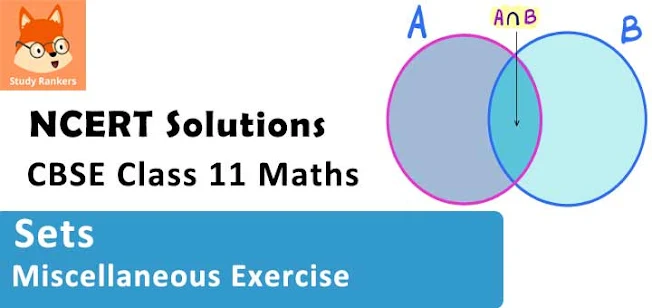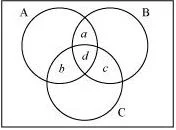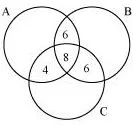# Class 11 Maths NCERT Solutions for Chapter 1 Sets Miscellaneous Exercise### Sets Exercise Miscellaneous Solutions

1. Decide, among the following sets, which sets are subsets of one and another:
A = {x: x ∈ R and x satisfy x2 – 8x + 12 = 0}, B = {2, 4, 6}, C = {2, 4, 6, 8…}, D = {6}.

Solution

A = {x: x ∈ R and x satisfies x2 – 8x + 12 = 0}
2 and 6 are the only solutions of x2 – 8x + 12 = 0.
∴ A = {2, 6}
B = {2, 4, 6}, C = {2, 4, 6, 8 …}, D = {6}
∴ D ⊂ A ⊂ B ⊂ C
Hence, A ⊂ B, A ⊂ C, B ⊂ C, D ⊂ A, D ⊂ B, D ⊂ C

2. In each of the following, determine whether the statement is true or false. If it is true, prove it. If it is false, give an example.
(i) If x ∈ A and A ∈ B, then x ∈ B
(ii) If A ⊂ B and B ∈ C, then A ∈ C
(iii) If A ⊂ B and B ⊂ C, then A ⊂ C
(iv) If A ⊄ B and B ⊄ C, then A ⊄ C
(v) If x ∈ A and A ⊄ B, then x ∈ B
(vi) If A ⊂ B and x ∉ B, then x ∉ A

Solution

(i) False
Let A = {1, 2} and B = {1, {1, 2}, {3}}
Now, 2 ∊ {1, 2} and {1, 2} ∊ {{3} , 1, {1, 2}}
∴ A ∊ B
However, 2 ∉ {{3}, 1, {1, 2}}

(ii) False
Let A = {2}, B = {0, 2} and C = {1, {0, 2}, 3}
As A ⊂ B
B ∊ C
However, A ∉ C

(iii) True
Let A ⊂ B and B ⊂ C.
Let x ∊ A
⇒ x ∊ B  [∵ A ⊂ B]
⇒ x ∊ C  [∵ B ⊂ C]
∴ A ⊂ C

(iv) False
Let A = {1, 2}, B = {0, 6,8}, and C = {0, 1, 2, 6, 9}
Accordingly, A⊄ B and B ⊄ C.
However, A ⊂ C

(v) False
Let A = {3, 5, 7} and B = {3, 4, 6}
Now, 5 ∊ A and A ⊄ B
However, 5 ∉ B

(vi) True
Let A ⊂ B and x ∉ B.
To show: x ∉ A
If possible, suppose x ∈ A.
Then, x ∈ B, which is a contradiction as x ∉ B
∴x ∉ A

3. Let A, B and C be the sets such that A ∪ B = A ∪ C and A ∩ B = A ∩ C. show that B = C.

Solution

Let, A, B and C be the sets such that A ∪ B = A ∪ C and A ∩ B = A ∩ C.
To show : B = C
Let x ∈ B
⇒ x ∈ A ∪ B  [B ⊂ A ∪ B]
⇒ x ∈ A ∪ C  [A ∪ B = A ∪ C]
⇒ x ∈ A or x ∈ C
Case I
x ∈ A
Also, x ∈ B
∴ x ∈ A ⋂ B
⇒ x ∈ A ∩ C [∵ A ∩ B = A ∩ C]
∴ x ∈ A and x ∈ C
∴ x ∈ C
∴ B ⊂ C
Similarly, we can show that C ⊂ B.
∴ B = C

4. Show that the following four conditions are equivalent:
(i) A ⊂ B
(ii) A – B = Φ
(iii) A ∪ B = B
(iv) A ∩ B = A

Solution

First, we have to show that (i) ⇔ (ii).
Let A ⊂ B
To show: A – B ≠ Φ
If possible, suppose A – B ≠ Φ
This means that there exists x ∈ A, x ≠ B, which is not possible as A ⊂ B.
∴ A – B = Φ
∴ A ⊂ B ⇒ A – B = Φ
Let A – B = Φ
To show: A ⊂ B
Let x ∈ A
Clearly, ∈ B because if x ∉ B, then A – B ≠ Φ
∴ A – B = Φ ⇒ A ⊂ B
∴ (i) ⇔ (ii)

Let A ⊂ B
To show : A ∪ B = B
Clearly, B ⊂ A ∪ B
Let x ∊ A ∪ B
⇒ x ∊ A or x ∊ B
Case I : x ∊ A
⇒ x ∊ B [∵ A ⊂ B]
∴ A ∪ B ⊂ B
Case II:  x ∊ B
Then, A ∪ B = B
Conversely, let A ∪ B = B
Let x ∊ A
⇒ x ∊ A ∪ B  [∵ A ⊂ A ∪ B]
⇒ x ∊ B  [∵ A ∪ B = B]
∴ A ⊂ B
Hence, (i) ⇔ (iii)
Now, we have to show that (i) ⇔ (iv) .

Let A ⊂ B
Clearly, A ∩ B ⊂ A

Let x ∊ A
We have to show that x ∊ A ∩ B
As A ⊂ B, x ∊ B
∴ x ∊ A ∩ B
∴ A ⊂ A ∩ B
Hence, A = A ∩ B
Conversely, suppose A ∩ B  = A

Let x ∊ A
⇒ x ∊ A ∩ B
⇒ x ∊ A and x ∊ B
⇒ x ∊ B
∴ A ⊂ B
Hence, (i) ⇔ (iv).

5. Show that if A ⊂ B, then C – B ⊂ C – A.

Solution

Let A ⊂ B
To show: C – B ⊂ C – A
Let x ∈ C – B
⇒ ∈ C and x ∉ B
⇒ x ∈ C and x ∉ A [A ⊂ B]
⇒ x ∈ C – A
∴ C – B ⊂ C – A

6. Assume that P (A) = P (B). Show that A = B.

Solution

Let P(A) = P(B)
To show: A = B
Let x ∈ A
A ∈ P(A) = P(B)
∴ ∈ C, for some C ∈ P(B)
Now, C ⊂ B
∴ x ∈ B
∴ A ⊂ B
Similarly, B ⊂ A
∴ A = B

7. Is it true that for any sets A and B, P (A) ∪ P (B) = P (A ∪ B)? Justify your answer.

Solution

False
Let A = {0, 1} and B = {1, 2}
∴ A ∪ B = {0, 1, 2}
P(A) = {Φ, {0}, {1}, {0, 1}}
P(B) = {Φ, {1}, {2}, {1, 2}}
P(A ∪ B) = {Φ, {0}, {1}, {2}, {0, 1}, {1, 2}, {0, 2}, {0, 1, 2}}
P(A) ∪ P(B) = {Φ, {0}, {1}, {0, 1}, {2}, {1, 2}}
∴ P(A) ∪ P(B) ≠ P(A ∪ B)

8. Show that for any sets A and B,
A = (A ∩ B) ∪ (A – B) and A ∪ (B – A) = (A ∪ B)

Solution

To show: A = (A ∩ B) ∪ (A – B)
Let x ∈ A
We have to show that x ∈ (A ∩ B) ∪ (A – B)
Case I:
x ∈ A ∩ B
Then, x ∈ (A ∩ B) ⊂ (A ∪ B) ∪ (A – B)
Case II:
x ∉ A ∩ B
⇒ x ∉ A or x ∉ B
∴ x ∉ B [x ∉ A]
∴ x ∉ A – B ⊂ (A ∪ B) ∪ (A – B)
∴ A ⊂ (A ∩ B) ∪ (A – B) …(1)
It is clear that
A ∩ B ⊂ A and (A – B) ⊂ A
∴ (A ∩ B) ∪ (A – B) ⊂ A …(2)
From (1) and (2), we obtain
A = (A ∩ B) ∪ (A – B)

To prove: A ∪ (B – A) ⊂ A ∪ B
Let x ∈ A ∪ (B – A)
⇒ x ∈ A or x ∈ (B – A)
⇒ x ∈ A or (x ∈ B and x ∉ A)
⇒ (x ∈ A or x ∈ B) and (x ∈ A or x ∉ A)
⇒ x ∈ (A ∪ B)
∴ A ∪ (B – A) ⊂ (A ∪ B) …(3)
Next, we show that (A ∪ B) ⊂ A ∪ (B – A).
Let y ∈ A ∪ B
⇒ y ∈ A or y ∈ B
⇒ (y ∈ A or y ∈ B) and (y ∈ A or y ∉ A)
⇒ y ∈ A or (y ∈ B and y ∉ A)
⇒ y ∈ A ∪ (B – A)
∴ A ∪ B ⊂ A ∪ (B – A) …(4)
Hence, from (3) and (4), we obtain A ∪ (B – A) = A ∪B.

9. Using properties of sets show that
(i) A ∪ (A ∩ B) = A

(ii) A ∩ (A ∪ B) = A.

Solution

(i) To show: A ∪ (A ∩ B) = A
We know that
A ⊂ A
A ∩ B ⊂ A
∴ A ∪ (A ∩ B) ⊂ A …(1)
Also, A ⊂ A ∪ (A ∩ B) …(2)
∴ From (1) and (2), A ∪ (A ∩ B) = A

(ii) To show: A ∩ (A ∪ B) = A
A ∩ (A ∪ B) = (A ∩ A) ∪ (A ∩ B)
= A ∪ (A ∩ B)
= A {from (1)}

10. Show that A ∩ B = A ∩ C need not imply B = C.

Solution

Let A = {0, 1}, B = {0, 2, 3}, and C = {0, 4, 5}
Accordingly, A ∩ B = {0} and A ∩ C = {0}
Here, A ∩ B = A ∩ C = {0}
However, B ≠ C  [2 ∈ B and 2 ∉ C]

11. Let A and B be sets. If A ∩ X = B ∩ X = Φ and A ∪ X = B ∪ X for some set X, show that A = B. (Hints A = A ∩ (A ∪ X), B = B ∩ (B ∪ X) and use distributive law)

Solution

Let A and B be two sets such that A ∩ X = B ∩ X = f and A ∪ X = B ∪ X for some set X.
To show: A = B
It can be seen that
A = A ∩ (A ∪ X) = A ∩ (B ∪ X) [A ∪ X = B ∪ X]
= (A ∩ B) ∪ (A ∩ X) [Distributive law]
= (A ∩ B) ∪ Φ [A ∩ X = Φ]
= A ∩ B …(1)
Now, B = B ∩ (B ∪ X)
= B ∩ (A ∪ X) [A ∪ X = B ∪ X]
= (B ∩ A) ∪ (B ∩ X) [Distributive law]
= (B ∩ A) ∪ Φ [B ∩ X = Φ]
= B ∩ A
= A ∩ B …(2)
Hence, from (1) and (2), we obtain A = B.

12. Find sets A, B and C such that A ∩ B, B ∩ C and A ∩ C are non-empty sets and A ∩ B ∩ C = Φ.

Solution

Let A = {0, 1}, B = {1, 2}, and C = {2, 0}.
Accordingly, A ∩ B = {1}, B ∩ C = {2}, and A ∩ C = {0}.
∴ A ∩ B, B ∩ C, and A ∩ C are non-empty.
However, A ∩ B ∩ C = Φ

13. In a survey of 600 students in a school, 150 students were found to be taking tea and 225 taking coffee, 100 were taking both tea and coffee. Find how many students were taking neither tea nor coffee?

Solution

Let U be the set of all students who took part in the survey.
Let T be the set of students taking tea.
Let C be the set of students taking coffee.
Accordingly, n(U) = 600, n(T) = 150, n(C) = 225, n(T ∩ C) = 100
To find: Number of student taking neither tea nor coffee i.e., we have to find n(T' ∩ C').
n(T' ∩ C') = n(T ∪ C)'
n(U) – n(T ∪ C)
n(U) – [n(T) + n(C) – n(T ∩ C)]
= 600 – [150 + 225 – 100]
= 600 – 275
= 325
Hence, 325 students were taking neither tea nor coffee.

14. In a group of students 100 students know Hindi, 50 know English and 25 know both. Each of the students knows either Hindi or English. How many students are there in the group?

Solution

Let U be the set of all students in the group.
Let E be the set of all students who know English.
Let H be the set of all students who know Hindi.
∴ H ∪ E = U
Accordingly, n(H) = 100 and n(E) = 50
n(H ∩ E) = 25
n(U) = n(H) + n(E) – n(H ∩ E)
100 + 50 – 25
= 125
Hence, there are 125 students in the group.

15. In a survey of 60 people, it was found that 25 people read newspaper H, 26 read newspaper T, 26 read newspaper I, 9 read both H and I,11 read both H and T, 8 read both T and I, 3 read all three newspapers. Find:
(i) the number of people who read at least one of the newspapers.
(ii) the number of people who read exactly one newspaper.

Solution

Let A be the set of people who read newspaper H.
Let B be the set of people who read newspaper T.
Let C be the set of people who read newspaper I.
Accordingly, n(A) = 25, n(B) = 26, and n(C) = 26
n(A ∩ C) = 9, n(A ∩ B) = 11, and n(B ∩ C) = 8
n(A ∩ B ∩ C) = 3
Let U be the set of people who took part in the survey.

(i) Accordingly,
n(A ∪ B ∪ C) = n(A) + n(B) + n(C) – n(A ∩ B) – n(B ∩ C) – n(C ∩ A) + n(A ∩ B ∩ C)
= 25 + 26 + 26 – 11 – 8 – 9 + 3
= 52
Hence, 52 people read at least one of the newspapers.

(ii) Let a be the number of people who read newspapers H and T only.Let b denote the number of people who read newspapers I and H only.
Let c denote the number of people who read newspapers T and I only.
Let d denote the number of people who read all three newspapers.
Accordingly, d = n(A ∩ B ∩ C) = 3
Now, n(A ∩ B) = a + d
n(B ∩ C) = c + d
n(C ∩ A) = b + d
∴ a + d + c + d + b + d = 11 + 8 + 9 = 28
⇒ a + b + c + d = 28 – 2d = 28 – 6 = 22
Hence, (52 – 22) = 30 people read exactly one newspaper.

16. In a survey it was found that 21 people liked product A, 26 liked product B and 29 liked product C. If 14 people liked products A and B, 12 people liked products C and A, 14 people liked products B and C and 8 liked all the three products. Find how many liked product C only.

Solution

Let A, B, and C be the set of people who like product A, product B, and product C respectively.
Accordingly, n(A) = 21, n(B) = 26, n(C) = 29, n(A ∩ B) = 14, n(C ∩ A) = 12,
n(B ∩ C) = 14, n(A ∩ B ∩ C) = 8
The Venn diagram for the given problem can be drawn asIt can be seen that number of people who like product C only is
{29 - (4 + 8 + 6)} = 11